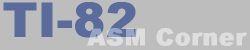Lesson 19
 TI-82 ASM Corner :: Programming Guide :: Lesson 19 Back | Home | Search

This lesson will give you some more in depth information on the way you can access data: addressing methods. So what exactly does addressing mean? Well, take for example LD A,(\$1234). Here, the data comes from a memory address. With LD A,(HL) the data also comes from a memory address, but this is register indirect addressing. It happens through the HL register.

The data is in one of the Z-80 registers or registerpairs.

 ``` Examples : INC HL LD A,B LD E,L DEC L ```

8-bits data is stored in the memory immediately after the opcode for the instruction

 ``` Examples : LD L,15 ADC A,125 SUB 20 CP 5 ```

Same as above, but the data is 16-bit this time

 ``` Examples : LD HL,\$800C LD IY,\$4414 LD IX,\$88B8 ```

Immediately after the opcode, there is a data word. A call or a jump needs to be made to that address or data needs to be received from that address.

 ``` Examples : JP \$0213 LD (\$800C),A LD HL,(\$8D24) LD A,(\$8000) ```

One of the register couples contains the address of the data
 ``` Examples : LD B,(HL) LD (DE),A LD (HL),\$0A RR (HL) JP (HL) ```

This way looks a lot like Register Indirect Addressing, but the Z-80 adds a byte (signed) to the address first. It only works with IX and IY.

 ``` Examples : SRL (IY-10) SET 3,(IY+05) ADD A,(IX+05) ```

To save time and memory, an address can sometimes be given with only 1 byte (signed). The real 2-byte address is then calculated starting from the Program Counter (Address=PC+2+offset).

 ``` Examples : JR 10 JR NC,-3 ```

The only page 0 addressing instructions on the Z-80 are the RST instructions. The address is calculated by putting the most significant bytes to \$00 and the least significant byte to the given one.

 ``` Examples : RST \$10 RST \$38 ```

 ``` Examples : SUB B EXX LDIR CPIR ```
 ``` Examples : BIT 3,(IY+\$05) SET 1,(HL) RES 5,D ```